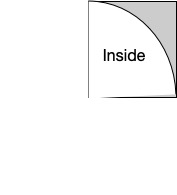# The Futility of Pi Denialism

To me, the strangest crackpots I’ve encountered through this blog are the π denialists.

When people have trouble with Cantor and differently sized infinities, I get it. It defies our intuitions. It doesn’t seem to make sense.

When you look at Gödel incompleteness theorem – it’s really hard to wrap your head around. It doesn’t seem to make sense. I get it.

When you talk about things like indescribable numbers, it’s crazy. How could it possibly be true? I get it.

But π?

It’s a pretty simple number: the ratio of the diameter of the circle and the circle’s circumference. There’s nothing all that difficult about what it means. And there are so many different ways of calculating it! We can use the nature of a circle, and derive series that compute it. We can write simple programs, do tactile demos, measure actual physical phenomena. And yet, there are people who fervently believe that it’s all a sham: that the value of π isn’t what we say it is. It’s exactly 4. Or it’s exactly 22/7. Or it’s exactly$\frac{4}{\phi^{\frac{1}{2}}}$. Or it’s not a number, it’s an acceleration.

It’s amazing. I constantly get mail – mostly from fans of either Jain (the author of the &phi;-based &pi; mentioned above), or from followers of Miles Mathis (he of “&pi; isn’t a ratio, it’s an acceleration” fame), insisting that I’m part of the great mathematical conspiracy to deny the true factual value of &pi;.

And yet… It’s so simple to demonstrate how wrong that is.

My favorite version is a simple program.

Here’s the idea, followed by the code.

• Take the unit square – the region of the graph from (0, 0) to (1, 1), and inside of it, an arc of the circle of radius 1 around (0,0).• Pick a random point, (x, y), anywhere inside of that square.
• If the distance from the origin ($x^2 + y^2$) is less than one, then the point is inside the circle. If it isn’t, then it’s outside of the circle.
• The probability,$p$, of any given random point being inside that circle is equal to the ratio of the area of the circle to the area of the square. The area of that region of the circle is:$\pi*1^2/4$, and the area of the the square is$1^2$. So the probability is$(1/4)\pi/1$, or$\pi/4$.
• So take a ton of random points, and count how many are inside the circle.
• The ratio of points inside the circle to total random points is$\pi/4$. The more random points you do this with, the closer you get to π.

We can turn that into a simple Python program:

from random import random

def computePi(points):
inside = 0
for i in range(points):
x = random()
y = random()
if (x*x + y*y) < 1.0:
inside = inside + 1
return (inside*1.0)/points * 4.0

for i in range(30):
pi = computePi(2**i)
print(f"Pi at 2**{i} iterations = {pi}")


The exact value that you’ll get when you run this depends on the random number generator, and the initial seed value. If you don’t specify a seed, most random number libraries will use something like last 32 digits of the current system time in nanoseconds, so you’ll get slightly different results each time you run it. I just ran it, and got:

Pi at 2**0 iterations = 4.0
Pi at 2**1 iterations = 4.0
Pi at 2**2 iterations = 3.0
Pi at 2**3 iterations = 2.0
Pi at 2**4 iterations = 3.5
Pi at 2**5 iterations = 2.75
Pi at 2**6 iterations = 3.0625
Pi at 2**7 iterations = 3.125
Pi at 2**8 iterations = 3.109375
Pi at 2**9 iterations = 3.1875
Pi at 2**10 iterations = 3.171875
Pi at 2**11 iterations = 3.126953125
Pi at 2**12 iterations = 3.12109375
Pi at 2**13 iterations = 3.14013671875
Pi at 2**14 iterations = 3.169677734375
Pi at 2**15 iterations = 3.1324462890625
Pi at 2**16 iterations = 3.14453125
Pi at 2**17 iterations = 3.147247314453125
Pi at 2**18 iterations = 3.138519287109375
Pi at 2**19 iterations = 3.1364669799804688
Pi at 2**20 iterations = 3.1443214416503906
Pi at 2**21 iterations = 3.141223907470703
Pi at 2**22 iterations = 3.141301155090332
Pi at 2**23 iterations = 3.1419320106506348
Pi at 2**24 iterations = 3.1415367126464844
Pi at 2**25 iterations = 3.1421539783477783
Pi at 2**26 iterations = 3.1420511603355408
Pi at 2**27 iterations = 3.1415300369262695
Pi at 2**28 iterations = 3.141532242298126
Pi at 2**29 iterations = 3.1415965482592583


I suspect that I could do a lot better using a special number library to reduce or eliminate the floating point roundoff errors, but I don’t really think it’s worth the time. Just this much, using a really simple, obvious, intuitive method produces a better result than any of the numbers pushed by the crackpots.

To support that previous statement: the best crackpot value for π is the one based on the golden ratio. That version insists that the true value of π is 3.14460551103. But you can see – by using the simple metric of counting points inside and outside the circle – that the actual value is quite different from that.

That’s what makes this breed of denialism so stupid. π isn’t complicated: it’s a simple ratio. And it’s easy to test using simple concepts. Pi relates the diameter (or radius) of a circle to the circumference or area of that circle. So any test that works with circles can easily show you what π is. There’s nothing mysterious or counterintuitive or debatable about it. It is what it is, and you can test it yourself.